LessonsA Gentle Introduction to Norwegian
Throwback Thursday
Learn Norwegian by looking at some of the most basic aspects of the language: greetings

Start Learning Norwegian in the next 30 Seconds with

|# Must-know Math Words and Phrases

Må-vite matte-ord og uttrykk.
20 Wordsmatte (n) fem
fem
math
Mitt favorittfag på skolen er matte.
My favorite subject in school is math.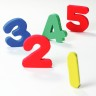nummer (n) neuter
neuter
number
Tallene 11 og 17 er begge primtall.
The numbers 11 and 17 are both prime numbers.odd
Tallet 11 er et eksempel på et oddetall.
The number 11 is an example of an odd number.neuter
even
Tallet 8 er et eksempel på et partall.
The number 8 is an example of an even number.pluss (n) neut
neut
plus
En pluss en er to.
One plus one is two.minus (n) neut
neut
minus
Åtte minus en er syv.
Eight minus one is seven.ganger (n) masc
masc
times
Tre ganger tre er ni.
Three times three is nine.dele (v)
divide
Ti delt på to er fem.
Ten divided by two is five.er lik (v)
equal
Fem pluss fem er lik ti.
Five plus five equals ten.punkt (n) neut
neut
point
ett punkt null tre
one point zero threeprosent (n) masc
masc
percent
Femti prosent av tolv er seks.
Fifty percent of twelve is six.rest (n) masc
masc
remainder
Hvis man deler fjorten på tre, er kvotienten fire med en rest på to.
If you divide fourteen by three, the quotient is four with a remainder of two.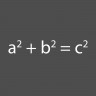formel (n) masc
masc
formula
Vet du formelen for volumet av en kule?
Do you know the formula for the volume of a sphere?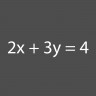ligning (n) masc
masc
equation
Studenten løste ligningen.
The student solved the equation.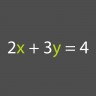variabel (n) masc
masc
variable
I matematiske problemer, er variablenee vanligvis representert med bokstavene "x" og "y".
In math problems, the variables are usually represented by the letters "x" and "y."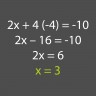løsning (n) masc
masc
solution
Hvis vi forenkler ligningen, er løsningen åpenbar.
If we simplify the equation, the solution is obvious.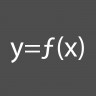funksjon (n) masc
masc
function
En funksjon representerer forholdet mellom to variabler.
A function represents the relationship between two variables.regne (v)
calculate
Kan du regne kostnadene for dagligvarene?
Can you calculate the cost of the groceries?fjerdedel (n) masc
masc
fourth
Når omgjort til en brøkdel, blir syttifem prosent tre fjerdedeler.
When converted into a fraction, seventy-five percent becomes three-fourths.halv (n) masc
masc
half
Oppskriften ba om en og en halv teskjeer sukker.
The recipe called for one and a half teaspoons of sugar.
None of our words match your filter
1 Comment
NorwegianClass101.com